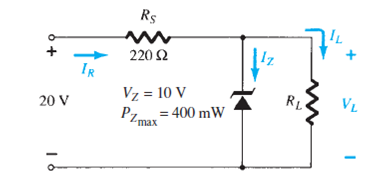Problem

# a. Determine VL, IL, IZ, and IR for the network of Fig. 2.186 if RL = 180 Ω.b. Repeat part...

a. Determine VL, IL, IZ, and IR for the network of Fig. 2.186 if RL = 180 Ω.

b. Repeat part (a) if RL = 470 Ω.

c. Determine the value of RL that will establish maximum power conditions for the Zener diode.

d. Determine the minimum value of RL to ensure that the Zener diode is in the “on” state.

FIG. 2.186#### Step-by-Step Solution

Solution 1

(a)

Refer to Figure $$2.186$$ in the textbook.

Calculate the load voltage, $$V_{L}$$.

$$V_{L}=\frac{R_{L} V_{i}}{R_{L}+R_{S}}$$

Here,

The load resistance $$R_{L}$$ is $$180 \Omega$$

The source resistance $$R_{S}$$ is $$220 \Omega$$

Voltage $$V_{i}$$ is $$20 \mathrm{~V}$$

Substitute $$180 \Omega$$ for $$R_{L}, 220 \Omega$$ for $$R_{S}$$ and $$20 \mathrm{~V}$$ for $$V$$ to obtain $$V_{L}$$ as follows:

\begin{aligned} V_{L} &=\frac{(180)(20)}{180+220} \\ &=\frac{3600}{400} \\ &=9 \mathrm{~V} \end{aligned}

Therefore, the load voltage, $$V_{L}$$ is $$9 \mathrm{~V}$$.

Here the load voltage $$V_{L}=9 \mathrm{~V}$$ is less than the ZENER voltage $$V_{Z}=10 \mathrm{~V} .$$ So, the diode does not conduct and hence the current through the diode is zero.

$$I_{Z}=0 \mathrm{~A}$$

Therefore, the value of the ZENER current, $$I_{Z}$$ is $$0 \mathrm{~A}$$.

Calculate the load current $$I_{L}$$.

\begin{aligned} I_{L} &=\frac{V_{i}}{R_{s}+R_{L}} \\ &=\frac{20}{180+220} \\ &=\frac{20}{400} \\ &=50 \mathrm{~mA} \end{aligned}

Therefore, the value of the load current $$I_{L}$$ is $$50 \mathrm{~mA}$$.

Calculate the reverse biased current $$I_{R}$$.

\begin{aligned} I_{R} &=I_{L} \\ &=50 \mathrm{~mA} \end{aligned}

Therefore, the value of the reverse biased current $$I_{R}$$ is $$50 \mathrm{~mA}$$.

(b)

Calculate the load voltage, $$V_{L}$$.

$$V_{L}=\frac{R_{L} V_{i}}{R_{L}+R_{S}}$$

Here,

The load resistance $$R_{L}$$ is $$470 \Omega$$

The source resistance $$R_{S}$$ is $$220 \Omega$$

Voltage $$V_{i}$$ is $$20 \mathrm{~V}$$

Substitute $$470 \Omega$$ for $$R_{L}, 220 \Omega$$ for $$R_{S}$$ and $$20 \mathrm{~V}$$ for $$V_{i}$$ in $$V_{L}$$.

\begin{aligned} V_{L} &=\frac{(470)(20)}{470+220} \\ &=\frac{9400}{690} \\ &=13.62 \mathrm{~V} \end{aligned}

Here the load voltage, $$V_{L}=13.62 \mathrm{~V}$$ is greater than the ZENER voltage, $$V_{Z}=10 \mathrm{~V} . \mathrm{So}$$, the diode conducts and the load voltage is equal to the ZENER voltage.

\begin{aligned} V_{L} &=V_{Z} \\ &=10 \mathrm{~V} \end{aligned}

Therefore, the load voltage, $$V_{L}$$ is $$10 \mathrm{~V}$$.

Calculate the current, $$I_{R_{\mathrm{s}}}$$.

\begin{aligned} I_{R_{s}} &=\frac{V_{R_{S}}}{R_{S}} \\ &=\frac{V_{i}-V_{L}}{R_{s}} \end{aligned}

Here,

Resistance $$R_{S}$$ is $$220 \Omega$$

Load voltage, $$V_{L}$$ is $$10 \mathrm{~V}$$

Voltage $$V_{i}$$ is $$20 \mathrm{~V}$$

Substitute $$220 \Omega$$ for $$R_{s}, 20 \mathrm{~V}$$ for $$V_{i}$$ and $$10 \mathrm{~V}$$ for $$V_{L}$$ in $$I_{R_{\mathrm{s}}}$$.

\begin{aligned} I_{R_{s}} &=\frac{20-10}{220} \\ &=45.45 \mathrm{~mA} \end{aligned}

Therefore, the current through the resistor, $$I_{R_{s}}$$ is $$45.45 \mathrm{~mA}$$.

Calculate the load Current, $$I_{L}$$.

\begin{aligned} I_{L} &=\frac{V_{L}}{R_{L}} \\ &=\frac{10}{470} \\ &=21.28 \mathrm{~mA} \end{aligned}

Therefore, the load Current, $$I_{L}$$ is $$21.28 \mathrm{~mA}$$.

Calculate the ZENER diode current, $$I_{Z}$$.

\begin{aligned} I_{Z} &=I_{R_{s}}-I_{L} \\ &=45.45 \mathrm{~mA}-21.28 \mathrm{~mA} \\ &=24.17 \mathrm{~mA} \end{aligned}

Therefore, the ZENER diode current $$I_{Z}$$ is $$24.17 \mathrm{~mA}$$.

(c)

Calculate the ZENER diode current, $$I_{Z}$$.

\begin{aligned} I_{Z} &=\frac{P_{\max }}{V_{Z}} \\ &=\frac{400 \mathrm{~mW}}{10 \mathrm{~V}} \\ &=40 \mathrm{~mA} \end{aligned}

Calculate the minimum load current $$I_{L_{\sin }}$$.

\begin{aligned} I_{L_{\min }} &=I_{R_{5}}-I_{Z_{\operatorname{mix}}} \\ &=45.45 \mathrm{~mA}-40 \mathrm{~mA} \\ &=5.45 \mathrm{~mA} \end{aligned}

Calculate the load resistance, $$R_{L}$$

\begin{aligned} R_{L} &=\frac{V_{L}}{I_{L_{\min }}} \\ &=\frac{10 \mathrm{~V}}{5.45 \times 10^{-3}} \\ &=1.835 \times 10^{3} \\ &=1.835 \mathrm{k} \Omega \end{aligned}

Therefore, the value of the load resistance, $$R_{L}$$ is $$1.835 \mathrm{k} \Omega$$

(d)

Calculate the load resistance $$R_{L}$$ when the ZENER diode is absent.

\begin{aligned} &V_{L}=\frac{R_{L} V_{i}}{R_{L}+R_{S}} \\ &10=\frac{20 R_{L}}{R_{L}+220} \\ &10 R_{L}+2200=20 R_{L} \\ &10 R_{L}=2200 \\ &R_{L}=220 \Omega \end{aligned}

Therefore, the load resistance $$R_{L}$$ is $$220 \Omega$$.